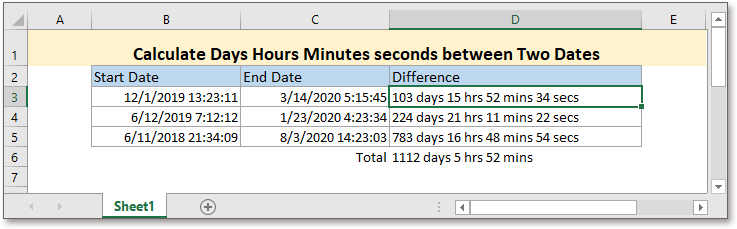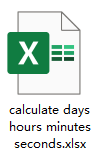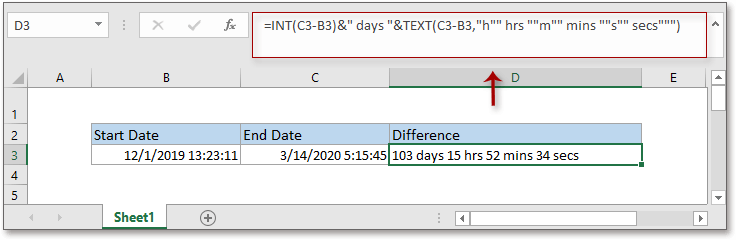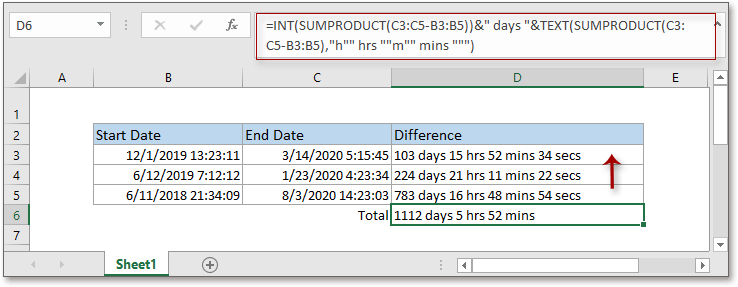## Excel Formula: Calculate Days Hours Minutes seconds between Two DatesGeneral in Excel, we use formulas to count the difference between two dates in days or difference between two times. However, in some special cases, we may want to get the days, hours, minutes, and seconds between two datetimes, this tutorial introduces the formula to solve this job.Generic formula:

 INT(end_datetime-start_datetime)&" days "&TEXT(end_datetime-start_datetime,"h"" hrs ""m"" mins ""s"" secs""")

Arguments

 End_datetime, start_datetime: the two dates that you to calculate the difference between.

Error

If the argument end_datetime is smaller than the argument start_datetime, the formula will return the error value #VALUE!.

Return value

This formula returns a text value.

How this formula work

To get the difference between two datetimes in cell B3 and cell C3, please use below formula:

 =INT(C3-B3)&" days "&TEXT(C3-B3,"h"" hrs ""m"" mins ""s"" secs""")

Press Enter key to get the result.Explanation

INT function: The INT function returns the integer part of a decimal number.

As the date is stored as serial whole number in Excel, and the time is stored as serial decimal number which is less than 1 in Excel, and the datetime is stored as a serial decimal number, for instance, the datetime 12/1/2019 13:23:11 is stored as 43800.5577662037 in Excel. Here the INT function extracts the whole number part of the difference between two datetimes to get the difference in days.

TEXT function: This function is used to convert number to text with a specified format.

&: This is a connector that connects the results of each part in the formula.

Tip: To get the total days, hours, minutes, and seconds between a set of start and end dates, you can adapt the formula using SUMPRODUCT function:

=INT(SUMPRODUCT(ends-starts))&" days "&TEXT(SUMPRODUCT(ends-starts),"h"" hrs ""m"" mins """)

The argument ends is the range of end dates, the starts is the cell range of start dates, for example, range C3:C5 contains the end dates, B3:B5 contains the start dates, please using the formula like this:

=INT(SUMPRODUCT(C3:C5-B3:B5))&" days "&TEXT(SUMPRODUCT(C3:C5-B3:B5),"h"" hrs ""m"" mins """)

Press Enter key.### The Best Office Productivity Tools

#### Kutools for Excel - Helps You To Stand Out From Crowd

Would you like to complete your daily work quickly and perfectly? Kutools for Excel brings 300 powerful advanced features (Combine workbooks, sum by color, split cell contents, convert date, and so on...) and save 80% time for you.

• Designed for 1500 work scenarios, helps you solve 80% Excel problems.
• Reduce thousands of keyboard and mouse clicks every day, relieve your tired eyes and hands.
• Become an Excel expert in 3 minutes. No longer need to remember any painful formulas and VBA codes.
• 30-day unlimited free trial. 60-day money back guarantee. Free upgrade and support for 2 years.#### Office Tab - Enable Tabbed Reading and Editing in Microsoft Office (include Excel)

• One second to switch between dozens of open documents!
• Reduce hundreds of mouse clicks for you every day, say goodbye to mouse hand.
• Increases your productivity by 50% when viewing and editing multiple documents.
• Brings Efficient Tabs to Office (include Excel), Just Like Chrome, Firefox, And New Internet Explorer.